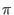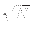Next: Bibliography Up: Quantum Computing and Grover's Previous: Implications on P =   Contents

# Conclusion

Quantum computation allows for exponential speed up and storage in a quantum register via quantum parallelism. The more basis states represented within the register, and hence the more speed up due to parallelism in the register, the more improbable it is that a desired state can be measured. Grover's algorithm handles this problem by relying on transformations which cause the amplitude of the marked state to increase at the expense of the non marked states, in a manner ways this is analogous to interference of waves.

Grover's algorithm is unique among quantum algorithms in that it shows a useful calculation that a quantum computer can calculate in fewer operations than any classical computer possibly can. At the heart of Grover's algorithm are two unitary transformations, the first is a selective phase inversion, which makes the sign of the amplitude of the target negative. The second unitary transformation is an inversion about average operation. Initially we place the amplitude of all states at the same positive value, each phase switch and inversion about average increases the amplitude of the target state. The exact number of times we perform these transformations is roughly/4for sufficiently large N. For a classical algorithm the best time bound is O(n).Next: Bibliography Up: Quantum Computing and Grover's Previous: Implications on P =   Contents
Matthew Hayward - Quantum Computing and Grover's Algorithm GitHub Repository